0
2013
Impact Factor

# On the Stability of Thomson’s Vortex $N$-gon and a Vortex Tripole/Quadrupole in Geostrophic Models of Bessel Vortices and in a Two-Layer Rotating Fluid: a Review

2019, Vol. 15, no. 4, pp.  533-542

Author(s): Kurakin L. G., Ostrovskaya I. V.

In this paper the two-layer geostrophic model of the rotating fluid and the model of Bessel vortices are considered. Kirchhoff's model of vortices in a homogeneous fluid is the limiting case of both of these models. Part of the study is performed for an arbitrary Hamiltonian depending on the distances between point vortices.
The review of the stability problem of stationary rotation of regular Thomson's vortex $N$-gon of identical vortices is given for ${N\geqslant 2}$. The stability problem of the vortex tripole/quadrupole is also considered. This axisymmetric vortex structure consists of a~central vortex of an arbitrary intensity and two/three identical peripheral vortices. In the model of a two-layer fluid, peripheral vortices belong to one of the layers and the central vortex can belong to either another layer or the same.
The stability of the stationary rotation is interpreted as orbital stability (the stability of a one-parameter orbit of a stationary rotation of a vortex system). The instability of the stationary rotation is instability of equilibrium of the reduced system. The quadratic part of the Hamiltonian and eigenvalues of the linearization matrix are studied.
The parameter space is divided into three parts: $\bf A$ is the domain of stability in an exact nonlinear setting, $\bf B$ is the linear stability domain, where the stability problem requires nonlinear analysis, and $\bf C$ is the instability domain.
In the stability problem of a vortex multipole, another definition of stability is used; it is the stability of an invariant three-parametric set of all trajectories of the families of stationary orbits. It is shown that in the case of non zero total intensity, the stability of the invariant set implies orbital stability.
Keywords: $N$-vortex problem, Thomson's vortex $N$-gon, point vortices, two-layer fluid, stability, Hamiltonian equation
Citation: Kurakin L. G., Ostrovskaya I. V., On the Stability of Thomson’s Vortex $N$-gon and a Vortex Tripole/Quadrupole in Geostrophic Models of Bessel Vortices and in a Two-Layer Rotating Fluid: a Review, Rus. J. Nonlin. Dyn., 2019, Vol. 15, no. 4, pp.  533-542
DOI:10.20537/nd190412

## References

 Borisov, A. V. and Mamaev, I. S., Mathematical Methods in the Dynamics of Vortex Structures, R&C Dynamics, Institute of Computer Science, Izhevsk, 2005, 368 pp. (Russian)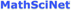Cabral, H. E. and Schmidt, D. S., “Stability of Relative Equilibria in the Problem of $N+1$ Vortices”, SIAM J. Math. Anal., 31:2 (1999/2000), 231–250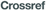Campbell, L. J., “Transverse Normal Modes of Finite Vortex Arrays”, Phys. Rev. A, 24:1 (1981), 514–534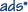Gryanik, V. M., “Dynamics of Singular Geostrophical Vortices in a $2$-Level Model of the Atmosphere (Ocean)”, Izv. Atmos. Ocean Phys., 19:3 (1983), 171–179; Izv. Akad. Nauk SSSR. Fiz. Atmos. Okeana, 19:3 (1983), 227–240 (Russian)Havelock, T. H., “The Stability of Motion of Rectilinear Vortices in Ring Formation”, Philos. Mag., 11:70 (1931), 617–633Kizner, Z., “Stability of Point-Vortex Multipoles Revisited”, Phys. Fluids, 23:6 (2001), 064104, 11 pp.Kizner, Z., “On the Stability of Two-Layer Geostrophic Point-Vortex Multipoles”, Phys. Fluids, 26:4 (2014), 046602, 18 pp.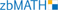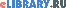Kurakin, L. G. and Yudovich, V. I., “The Stability of Stationary Rotation of a Regular Vortex Polygon”, Chaos, 12:3 (2002), 574–595Kurakin, L. G., Ostrovskaya, I. V., and Sokolovskiy, M. A., “Stability of Discrete Vortex Multipoles in Homogeneous and Two-Layer Rotating Fluid”, Dokl. Phys., 60:5 (2015), 217–223; Dokl. Akad. Nauk, 462:2 (2015), 161–167 (Russian)Kurakin, L. G., Ostrovskaya, I. V., and Sokolovskiy, M. A., “On the Stability of Discrete Tripole, Quadrupole, Thomson' Vortex Triangle and Square in a Two-Layer/Homogeneous Rotating Fluid”, Regul. Chaotic Dyn., 21:3 (2016), 291–334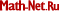Kurakin, L. G. and Ostrovskaya, I. V., “On Stability of the Thomson's Vortex $N$-Gon in the Geostrophic Model of the Point Bessel Vortices”, Regul. Chaotic Dyn., 22:7 (2017), 865–879Kurakin, L. G., Lysenko, I. A., Ostrovskaya, I. V., and Sokolovskiy, M. A., “On Stability of the Thomson's Vortex $N$-Gon in the Geostrophic Model of the Point Vortices in Two-Layer Fluid”, J. Nonlinear Sci., 29:4 (2019), 1659–1700Mertz, G., “Stability of Body-Centered Polygonal Configurations of Ideal Vortices”, Phys. Fluids, 21:7 (1978), 1092–1095Morikawa, G. K. and Swenson, E. V., “Interacting Motion of Rectilinear Geostrophic Vortices”, Phys. Fluids, 14:6 (1971), 1058–1073Thomson, W., “Floating Magnets (Illustrating Vortex Systems)”, Nature, 18 (1878), 13–14; Kelvin, W. T., Mathematical and Physical Papers, v. 4, Cambridge Univ. Press, Cambridge, 1910, 162–164
 Thomson, J. J., Treatise on the Motion of Vortex Rings, Macmillan, London, 1883, 156 pp.Sokolovskiy, M. A. and Verron, J., “Some Properties of Motion of $A + 1$ Vortices in a Two-Layer Rotating Fluid”, Nelin. Dinam., 2:1 (2006), 27–54 (Russian)Sokolovskiy, M. A. and Verron, J., Dynamics of Vortex Structures in a Stratified Rotating Fluid, Atmos. Oceanogr. Sci. Libr., 47, Springer, Cham, 2014, XII, 382 pp.Stewart, H. J., “Periodic Properties of the Semi-Permanent Atmospheric Pressure Systems”, Quart. Appl. Math., 1 (1943), 262–267Stewart, H. J., “Hydrodynamic Problems Arising from the Investigation of the Transverse Circulation in the Atmosphere”, Bull. Amer. Math. Soc., 51 (1945), 781–799